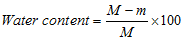# water content

(redirected from Volumetric moisture content)
Also found in: Encyclopedia.

## water content

Water in a contact lens expressed as a percentage of the total mass of the lens in its hydrated state under equilibrium conditions with physiological saline solution containing 9 g/l sodium chloride at a temperature of 20 ± 0.5ºC and with a stated pH value.where M is the mass of hydrated lens, m is the mass of dry lens.The US Food and Drug Administration (FDA) has categorized hydrogel contact lenses into four groups according to their water content and their surface reactivity (referred to as ionic if it contains more than 0.2% ionic material, and nonionic otherwise). Group 1: water content less than 50% and non-ionic. Group 2: water content greater than 50% and non-ionic. Group 3: water content less than 50% and ionic. Group 4: water content greater than 50% and ionic.
References in periodicals archive ?
The results of varying the volumetric moisture content of the soil and the thickness of the insulation and soil layers are evaluated to determine their impact on the thermal performance of the build-up in maintaining a target center of soil temperature.
The variation of the deformation with volumetric moisture content can be conversed by the test results.
It also highlights the differences between gravimetric and volumetric moisture content. The sensors predict the moisture content based on electrical conductivity measurements.
In this case, the lower values of the measured values of the volumetric moisture content W in emulsions of the <<nonpolar liquid dielectric-water>> type can be from 10 [cm.sup.3]/[m.sup.3] for transformer oil up to 100 [cm.sup.3]/[m.sup.3] for aviation kerosene.
where [theta] is the volumetric moisture content ([cm.sup.3] *-[cm.sup.-3]), D([theta]) is the diffusion coefficient ([cm.sup.2]x[mm.sup.-1]), x is the distance from the water inlet (cm), [[theta].sub.a] and [[theta].sub.0] are, respectively, the initial volumetric moisture content of soil sample and the volumetric moisture content at water inlet ([cm.sup.3]x[cm.sup.-3]), and t is the infiltration time (min).
In the growing season of 2013 and 2014, the surface volumetric moisture content was collected and measured by a Theta moisture sensor every day from June 11 to July 3.
(2006) monitored the ECa and volumetric moisture content of soil from June 1999 to October 2000 and concluded that ECa is strongly influenced by soil moisture content.
The volumetric moisture content of soil samples used in the uniaxial compression test to obtain the compression curve is shown in Table 3.
Comparison of the gravimetric and volumetric moisture content in the field with structure score 2 (bulk density average 1.12, s.d.
Volumetric moisture content of soil varied significantly among sampling sites and among landscape positions under different land-use/land covers (Figure 2).
For a same value of travel time ([mu]sec), higher volumetric moisture content was found in undisturbed samples than in disturbed samples (Figure 3), which is due to two factors: grain size and bulk density.
In the second stage of the experimental studies were identified value of volumetric moisture content of the prepared mixtures <<transformer oil--water>> in the moisture content range (10 - [10.sup.4]) [cm.sup.3]/[m.sup.3].

Site: Follow: Share:
Open / Close